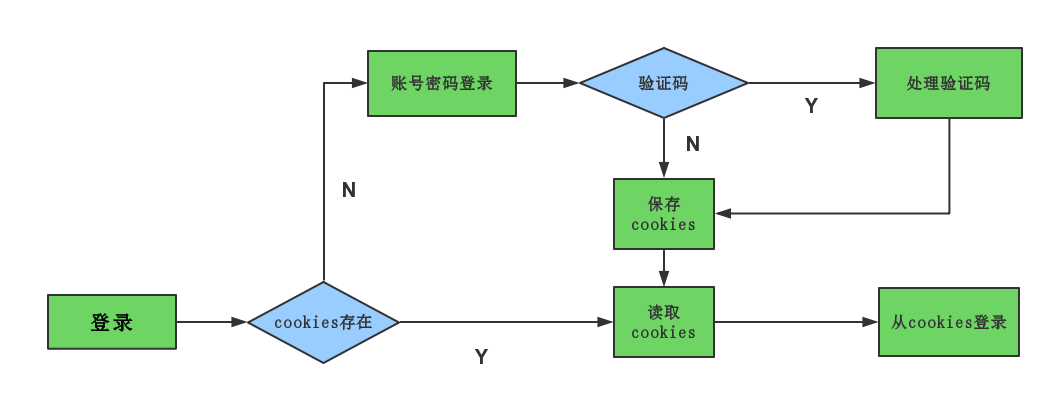QQ 空间爬虫之模拟登录
Mar 24, 2017

#### 1. 登录流程#### 2. 常规登录

``````def login(self):
exc = None
while True:
try:
if exc is None:
break
else:
verifier = exc.verifier
open('verify.jpg', 'wb').write(verifier.fetch_image())
print('saved verify.jpg')
vcode = input('input verify:')
verifier.verify(vcode)
exc = None
except qqlib.NeedVerifyCode as e:
if e.message != None:
print e.message
exc = e
``````

``````def save_cookie_to_file(cookie, cookie_file):
``````

``````def load_cookie_from_file(cookie_file):
return None
``````
##### 3.3 `cookiejar` 对象转字符串

``````def cookiejar_to_string(cookies):
return None
else:
cookie += keys+ '=' + values + ';'
``````

#### 4. `g_tk` 值

``````def g_tk(self):
h = 5381
for c in s:
h += (h << 5) + ord(c)
return h & 0x7fffffff
``````

#### 5. 检查登录

``````https://h5.qzone.qq.com/proxy/domain/r.qzone.qq.com/cgi-bin/user/cgi_personal_card?uin=用户qq&g_tk=g_tk值
``````

Back to posts